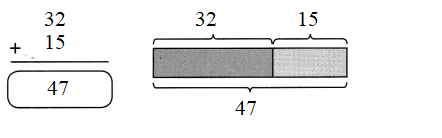# Math in Focus Grade 2 Chapter 10 Practice 1 Answer Key Meaning of Sum

Practice the problems of Math in Focus Grade 2 Workbook Answer Key Chapter 10 Practice 1 Meaning of Sum to score better marks in the exam.

## Math in Focus Grade 2 Chapter 10 Practice 1 Answer Key Meaning of Sum

Find the sum of the numbers.

96 and 73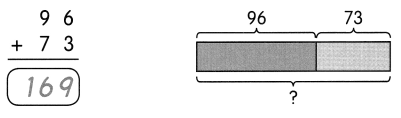The sum of 96 and 73 is 169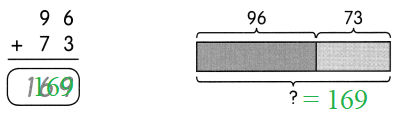Question 1.
700 and 200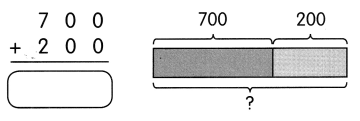The sum of ___ and ___ is ____.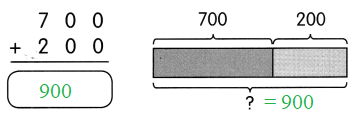The sum of 700 and 200 is 900

Question 2.
215 and 507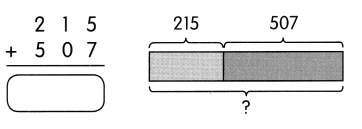The sum of ___ and ___ is ____.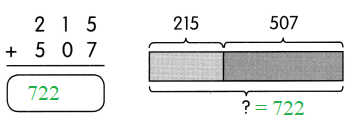The sum of 215 and 507 is 722

Solve.

Question 3.
Al spent $27 on a shirt and$120 on books. Find the sum of money Al spent.
___ + ___ = _____
The sum of money Al spent is $_____. Answer: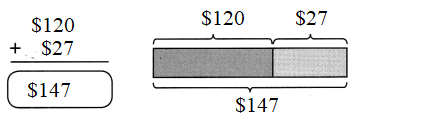Explanation: Given: Al has spent$ 27 on a shirt
And $120 on a Book The total amount he spent was$27 + $120 =$147
The sum of money Al spent is \$ 147.

Question 4.
Harry is 12 years old. His sister is 9 years younger. Find the sum of their ages.
____ + ___ = ____
The sum of their ages is ____ years.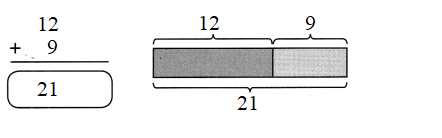Explanation:
Given:
harry is 12 years old
and he has a sister who is 9 years old
the total sum of the there age will be as follows
12 + 9 = 21
The sum of their ages is 21 years.

Question 5.
Greg has collected 32 green jumping beans. His mother gives him 15 red jumping beans more. Find the sum of jumping beans Greg has now.
___ + ____ = ____
The sum of jumping beans Greg has now is ____